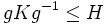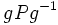# Pi-dominating pi-subgroup implies pi-Hall

This article gives a proof/explanation of the equivalence of multiple definitions for the term order-dominating Hall subgroup
View a complete list of pages giving proofs of equivalence of definitions

## Statement

Suppose$G$ is a finite group,$H$ is a subgroup of$G$, and$\pi$ is a set of primes such that:

• The set of prime divisors of the order of$H$ is in$\pi$.
• Given any$\pi$-subgroup$K$ of$G$ (i.e., any subgroup for which all prime divisors of its order are in$\pi$), there exists$g \in G$ such that$gKg^{-1} \le H$.

Then,$H$ is a$\pi$-Hall subgroup: in particular, its index is relatively prime to$\pi$, or equivalently, its order is the unique largest$\pi$-number dividing the order of$G$.

Note that this also shows that a subgroup is$\pi$-dominating for a set of primes$\pi$ iff it is an order-dominating Hall subgroup.

## Proof

Given: A finite group$G$, a$\pi$-dominating subgroup$H$.

To prove:$H$ is$\pi$-Hall.

Proof: It suffices to show that for every$p \in \pi$, the largest power of$p$ dividing the order of$G$ also divides the order of$H$.

For this, let$P$ be a$p$-Sylow subgroup (existence follows from fact (1)). The order of$P$ is the largest power of$p$ dividing the order of$G$. By the assumption, some conjugate$gPg^{-1}$ is in$H$. The order of$gPg^{-1}$ equals the order of$P$, and by fact (2), this divides the order of$H$. Thus, the order of$H$ is a multiple of the largest power of$p$ dividing the order of$G$, and this completes the proof.